Next: Pyramidal Algorithm with one Up: Pyramidal Algorithm Previous: Pyramidal Algorithm

### The Laplacian Pyramid

The Laplacian Pyramid has been developed by Burt and Adelson in 1981  in order to compress images. After the filtering, only one sample out of two is kept. The number of pixels decreases by a factor two at each scale.

The convolution is done with the filter h by keeping one sample out of two (see figure 14.7):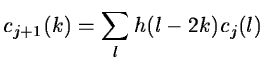(14.38)To reconstruct cj from cj+1, we need to calculate the difference signal wj+1.(14.39)

whereis the signal reconstructed by the following operation (see figure 14.8):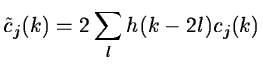(14.40)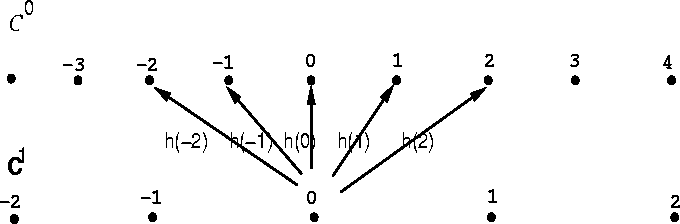In two dimensions, the method is similar. The convolution is done by keeping one sample out of two in the two directions. We have:(14.41)

and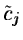is:(14.42)

The number of samples is divided by four. If the image size is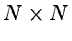, then the pyramid size is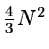. We get a pyramidal structure (see figure 14.9).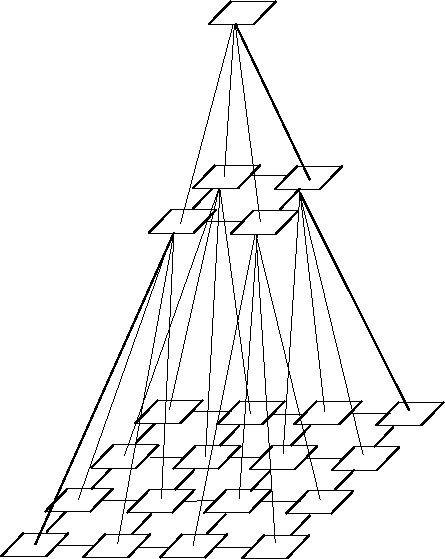The laplacian pyramid leads to an analysis with four wavelets  and there is no invariance to translation.Next: Pyramidal Algorithm with one Up: Pyramidal Algorithm Previous: Pyramidal Algorithm
Petra Nass
1999-06-15